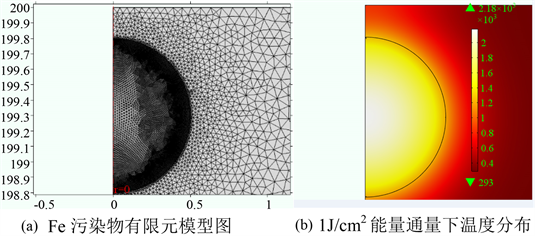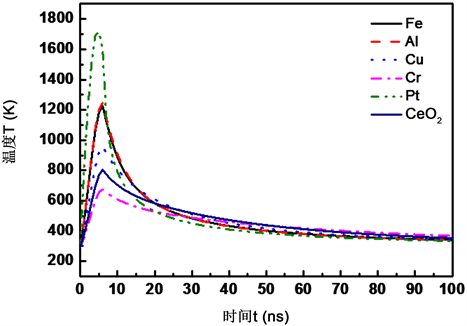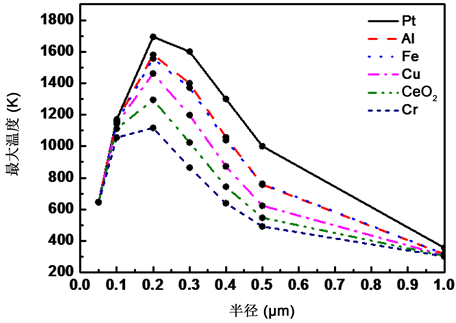#### 期刊菜单

Simulation Study on the Effect of Solid Contaminants on the Laser-Induced Hot Damage of Al2O3
DOI: 10.12677/CMP.2019.83009, PDF , HTML, XML, 下载: 779  浏览: 1,434  国家自然科学基金支持

Abstract: Al2O3 is the most widely used in high power laser material, the surface damage at work can cause serious decline, optical device performance is the main factor restricting the development of high functional laser device further. One of the surface damages is mainly caused by the surface at-tachment of contaminants, among them, the solid contaminants are the main source of induced damage, therefore, research on the surface of Al2O3 solid contaminants laser damage resistance of the impact is particularly important. Effect on surface of Al2O3 based on solid inorganic contami-nants was studied, with different types, different size of the surface of the solid pollutants of Al2O3 are simulated to examine the effects of temperature, and found that when Al2O3 surface contami-nants, laser damage is more likely to occur, and different types, different sizes of contaminants have different effects on Al2O3 laser damage.

1. 引言

2. 污染物的来源与分类

3. 污染物对温度场影响的理论模型

$\frac{1}{{D}_{i}}\frac{\partial {T}_{i}}{\partial t}=\frac{1}{{r}^{2}}\frac{\partial }{\partial r}\left({r}^{2}\frac{\partial {T}_{i}}{\partial r}\right)+\frac{A}{{k}_{i}}$$r (1)

$\frac{1}{{D}_{s}}\frac{\partial {T}_{s}}{\partial t}=\frac{1}{{r}^{2}}\frac{\partial }{\partial r}\left({r}^{2}\frac{\partial {T}_{s}}{\partial r}\right)$$r>a$ (2)

Ti，Ts——污染物温度，基底温度；

t——时间；

ρi，ρs——污染物密度，基底密度；

Ci，Cs——污染物质量热容，基底质量热容；

ki，ks——污染物导热系数，基底导热系数；

r——距离激光光斑中心距离；

a——球形污染物半径；

A——热源项。

$A=\left\{\begin{array}{l}\frac{3QI}{4{a}^{3}}\text{\hspace{0.17em}}\text{\hspace{0.17em}}\text{\hspace{0.17em}}\text{\hspace{0.17em}}\text{\hspace{0.17em}}0{t}_{p}\end{array}$ (3)

$Q=p\frac{16{\pi }^{2}{a}^{3}k}{\lambda }\underset{0}{\overset{\pi /2}{\int }}\mathrm{sin}\theta {\left(\mathrm{cos}\theta \right)}^{3}\text{d}\theta$ (4)

I——入射激光功率密度；

tp——激光脉冲宽度；

k——污染物的消光系数；

p——散射系数。

${T}_{i}={T}_{s}={T}_{0},\text{\hspace{0.17em}}\text{\hspace{0.17em}}\forall r,t=0$ (5)

${T}_{s}={T}_{0},\text{\hspace{0.17em}}\text{\hspace{0.17em}}\forall t,r\to \infty$ (6)

${T}_{i}={T}_{s},\text{\hspace{0.17em}}\text{\hspace{0.17em}}{k}_{i}\frac{\partial {T}_{i}}{\partial r}={k}_{s}\frac{\partial {T}_{s}}{\partial r},\text{\hspace{0.17em}}\text{\hspace{0.17em}}r=a$ (7)

$T=\frac{{a}^{2}A}{{k}_{i}}\left[\frac{1}{3}\frac{{k}_{i}}{{k}_{s}}+\frac{1}{6}\left(1-\frac{{r}^{2}}{{a}^{2}}\right)-\frac{2aB}{r\pi }\underset{0}{\overset{\infty }{\int }}\text{ }\text{ }{\text{e}}^{-{x}^{2}\frac{{t}_{p}}{r}}\frac{\left(\mathrm{sin}x-x\mathrm{cos}x\right)\mathrm{sin}\left(\frac{rx}{a}\right)}{{x}^{2}\left({\left(C\mathrm{sin}x-x\mathrm{cos}x\right)}^{2}+{B}^{2}{x}^{2}{\left(\mathrm{sin}x\right)}^{2}\right)}\text{d}x\right],\text{\hspace{0.17em}}\text{\hspace{0.17em}}0

$T=\frac{{a}^{3}A}{E{k}_{i}}\left[\frac{1}{3}\frac{{k}_{i}}{{k}_{s}}-\frac{2}{\pi }\underset{0}{\overset{\infty }{\int }}\text{ }\text{ }{\text{e}}^{-\frac{{x}^{2}{t}_{p}}{r}}\frac{\left(\mathrm{sin}x-x\mathrm{cos}x\right)\left[Bx\mathrm{sin}x\mathrm{cos}Fx-\left(C\mathrm{sin}x-x\mathrm{cos}x\right)\mathrm{sin}Fx\right]}{{x}^{3}\left({\left(C\mathrm{sin}x-x\mathrm{cos}x\right)}^{2}+{B}^{2}{x}^{2}{\left(\mathrm{sin}x\right)}^{2}\right)}\text{d}x\right],\text{\hspace{0.17em}}\text{\hspace{0.17em}}r>a$

(8)

4. 仿真分析

4.1. 污染物对温度分布影响的仿真分析

4.1.1. 污染物种类对温度分布的影响Figure 1. Fe calculation model and temperature simulation resultsFigure 2. Comparison of temperature at different pollutant boundary

4.1.2. 污染物尺寸对温度分布的影响Figure 3. Comparison of the maximum temperature of different contaminants irradiated by 0.5 J/cm2 laser at different radia

5. 结论

  张林, 杜凯. 激光惯性约束聚变靶技术现状及其发展趋势[J]. 强激光与粒子束, 2013, 25(12): 3091-3097.  刘成安, 师学明. 美国激光惯性约束聚变能源研究综述[J]. 原子核物理评论, 2013, 30(1): 89-93.  Peng, H., Zhang, X.M. and Wei, X. (2008) Status of the SG-III Solid State Laser Project. Proceedings of SPIE, 3492, 32009.  Kalantar, D., Nelson, D. and Bliss, E. (2011) National Ignition Facility System Alignment. Applied Optics, 50, 1136-1157. https://doi.org/10.1364/AO.50.001136  Horvath, J.A. (1996) NIF/LMJ Prototype Amplifier Mechanical Design. Lawrence Livermore National Laboratory, 148-157. https://doi.org/10.1117/12.294298  Schweyen, J.C. (1998) Ghost Reflection Analysis for the Main Laser of the National Ignition Facility. Proceedings of SPIE, 3482, 748-753. https://doi.org/10.1117/12.321973  李良钰, 王仕, 李银柱. “神光”装置中空间滤波器的鬼点分析[J]. 中国激光, 2001, 28(9): 826-828.  王方, 朱启华, 张清泉. 高功率激光装置中透镜一阶“鬼”点形成规律分析[J]. , 2005, 29(3): 170-171.  Feit, A. and Rubenchik, A.M. (2003) Influence of Subsurface Cracks on Laser-Induced Surface Damage. Proceeding of SPIE, 5273, 265-273.  Stuart, B.C., Feit, M.D. and Herman, S. (1996) Nanosecond-to-Femtosecond Laser-Induced Breakdown in Dielectrics. Physical Review B Condensed Matter, 53, 1749-1756. https://doi.org/10.1103/PhysRevB.53.1749  Hopper, R.W. and Uhlmann, D.R. (1970) Mechanism of Inclusion Damage in Laser Glass. Journal of Applied Physics, 41, 4023-4037. https://doi.org/10.1063/1.1658407  Martin, P., Moroño, A. and Hodgson, E.R. (2004) Laser Induced Damage Enhancement Due to Stainless Steel Deposition on KS-4V and KU1 Quartz Glasses. Journal of Nuclear Materials, 329, 1442-1445. https://doi.org/10.1016/j.jnucmat.2004.04.162  Honig, J., Norton, M.A. and Johnson, M.A. (2005) Experimental Study of 351-nm and 527-nm Laser-Initiated Surface Damage on Fused Silica Surfaces Due to Typical Contaminants. Proceedings of SPIE, 5647, 129-135. https://doi.org/10.1117/12.597314  Génin, F.Y., Feit, M.D. and Kozlowski, M.R. (2000) Rear-Surface Laser Damage on 355-nm Silica Optics Owing to Fresnel Diffraction on Front-Surface Contamination Particles. Applied Optics, 39, 3654-3663. https://doi.org/10.1364/AO.39.003654  Feit, M.D., Rubenchik, A.M. and Faux, D.R. (1996) Modeling of Laser Damage Initiated by Surface Contamination. Proceedings of SPIE, 2966, 417-424. https://doi.org/10.1117/12.274234  Koldunov, M.F., Manenkov, A.A. and Pocotilo, I.L. (1994) Theory of Laser-Induced Damage to Optical Coatings: Inclusion-Initiated Thermal Explosion Mechanism. Proceedings of SPIE-The International Society for Optical Engineering, 2114. https://doi.org/10.1117/12.180892